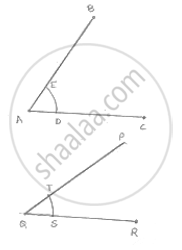# Draw an Angle and Label It as ∠Bac. Construct Another Angle, Equal to ∠Bac. - Mathematics

Draw an angle and label it as ∠BAC. Construct another angle, equal to ∠BAC.

#### SolutionSteps of construction:
1. Draw an angle ABO and a Line segment QR
2. With center A and any radius, draw an arc which intersects ∠BAC at E and O
3. With center Q and same radius draw arc which intersect QR at S.
4. With center S and radius equal to DE, draw an arc which intersect previous arc at T
5. Draw a line segment joining Q and T

∴ ∠PQR =  ∠BAC

Concept: Basic Constructions
Is there an error in this question or solution?

#### APPEARS IN

RD Sharma Mathematics for Class 9
Chapter 16 Constructions
Exercise 16.2 | Q 1 | Page 9

Share